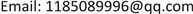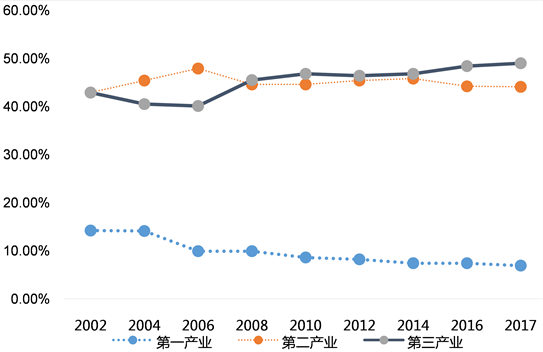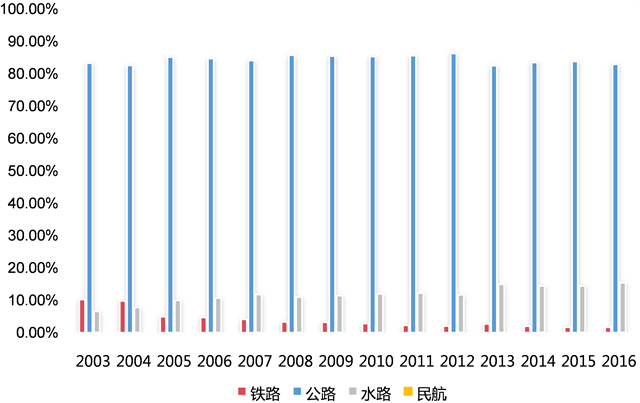﻿ 重庆市交通运输结构与产业结构的关联分析 The Association Analysis of Transportation Structure and Industrial Structure in Chongqing

Modern Management
Vol. 08  No. 04 ( 2018 ), Article ID: 26485 , 6 pages
10.12677/MM.2018.84053

The Association Analysis of Transportation Structure and Industrial Structure in Chongqing

Hong Xiao1, Qingrui Zhang1, Yanjuan Ren1, Bo Hang2

1Department of Economics and Management, Chongqing Jiaotong University, Chongqing

2Hubei University of Arts and Science, Xiangyang HubeiReceived: Jul. 31st, 2018; accepted: Aug. 13th, 2018; published: Aug. 20th, 2018ABSTRACT

The national economy is closely related to the transportation structure and the adjustment of industrial structure affects the development of transportation structure. Taking Chongqing for example, some conclusions can be drawn from analyzing the development of industrial structure, transportation structure and the grey relational theory. According to the grey correlation theory, water transport is closely related to highway in the transportation of goods, which means that if the corresponding measures are not taken, water transport will be replaced by highway. Therefore, Chongqing’s various modes of transportation should be coordinated with each other according to its own characteristics in the future.

Keywords:Industrial Structure, Transport Structure, Grey Relation

1重庆交通大学经济与管理学院，重庆

2湖北文理学院，湖北 襄阳1. 引言

2. 重庆市产业结构规模及其变化

2.1. 重庆市地区生产总值增长情况

2.2. 重庆市产业结构调整情况

3. 重庆市交通运输发展状况

3.1. 重庆市交通运输总量的变化Figure 1. Industrial structure ratio over the years in ChongqingTable 1. Passenger and cargo transportation and turnover over the years in Chongqing

3.2. 重庆市交通运输结构变动状况

4. 重庆市产业结构与交通运输结构的定量分析

4.1. 重庆市产业结构与交通运输结构关联度分析

1) 对参考数列和比较数列进行无量纲化处理Figure 2. Proportion of freight volume structure over the years in Chongqing

${x}_{i}\left(k\right)=\frac{{x}_{i}\left(k\right)-\mathrm{min}{x}_{i}\left(k\right)}{\mathrm{max}{x}_{i}\left(k\right)-\mathrm{min}{x}_{i}\left(k\right)}$

2) 求参考数列与比较数列的灰色关联系数 ${\xi }_{i}\left(k\right)$

${\xi }_{i}\left(k\right)=\frac{\begin{array}{c}\mathrm{min}\mathrm{min}\\ i\text{}k\end{array}|{x}_{0}\left(k\right)-{x}_{i}\left(k\right)|+\rho \begin{array}{c}\mathrm{min}\mathrm{max}\\ i\text{}k\end{array}|{x}_{0}\left(k\right)-{x}_{i}\left(k\right)|}{|{x}_{0}\left(k\right)-{x}_{i}\left(k\right)|+\rho \begin{array}{c}\mathrm{max}\mathrm{max}\\ i\text{}k\end{array}|{x}_{0}\left(k\right)-{x}_{i}\left(k\right)|}$

3) 通过计算，得出参考数列与比较数列的关联系数。由于关联系数 ${\xi }_{i}\left(k\right)$ 较多，信息过于分散，不便于整体性比较，因此有必要将各个时刻的关联系数集中为一个值，取其平均值，称之为关联度。

${r}_{i}=\frac{1}{N}\sum _{k-1}^{N}{\xi }_{i}\left(k\right)$

3) 通过计算得到2008年至2016年8年间重庆市产业结构与交通运输之间的灰关联度，如表2所示。

4.2. 重庆市交通运输结构灰色斜率关联度分析Table 2. Relation between industrial structure and transportation structure in ChongqingTable 3. Gray slope correlation between freight turnover of three modes of transport

5. 结论

The Association Analysis of Transportation Structure and Industrial Structure in Chongqing[J]. 现代管理, 2018, 08(04): 435-440. https://doi.org/10.12677/MM.2018.84053

1. 1. 李伟达. 交通运输结构发展演变规律的理论分析[J]. 水运管理, 2000(7): 3-7.

2. 2. 彭湘涛. 交通运输对产业结构的影响研究[D]: [硕士学位论文]. 长沙: 长沙理工大学, 2009.

3. 3. 李崇良. 西宁市产业结构对交通运输影响研究[D]: [硕士学位论文]. 青海: 青海师范大学, 2011.

4. 4. 聂正英. 产业结构对交通运输需求影响的定量分析[D]: [硕士学位论文]. 北京: 北京交通大学, 2010.

5. 5. 初克波. 交通运输与产业结构的灰色系统关联模式的综合分析[J]. 交通运输工程与信息学报, 2009(9): 3-7.

6. 6. 冯旭杰, 孙全欣, 钱堃, 刘明君. 区域综合交通运输需求与产业结构的协整关系分析[J]. 交通运输系统工程与信息, 2012(12): 6-12.

7. 7. 吴峰, 施其洲. 基于熵值理论的产业结构与交通运输结构关系研究[J]. 交通运输系统工程与信息, 2006(2): 1-6.